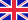•# Simplex algorithm

Autor: Lambert M. Surhone

n mathematical optimization theory, the simplex algorithm, created by the American mathematician George Dantzig in 1947, is a popular algorithm for numerically solving linear programming problems. The journal Computing in Science and Engineering listed it... Viac o knihe

Na objednávku, dodanie 2-4 týždne

25.42 €

bežná cena: 29.90 €

## O knihe

n mathematical optimization theory, the simplex algorithm, created by the American mathematician George Dantzig in 1947, is a popular algorithm for numerically solving linear programming problems. The journal Computing in Science and Engineering listed it as one of the top 10 algorithms of the century. The method uses the concept of a simplex, which is a polytope of N + 1 vertices in N dimensions: a line segment in one dimension, a triangle in two dimensions, a tetrahedron in three-dimensional space and so forth.

• Vydavateľstvo: Betascript Publishers
• Rok vydania: 2009
• Formát: Paperback
• Rozmer: 220 x 150 mm
• Jazyk: Anglický jazyk
• ISBN: 9786130302320

Generuje redakčný systém BUXUS CMS spoločnosti ui42.